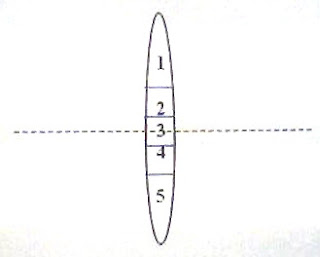## Pages

`“Life is like riding a bicycle.  To keep your balance you must keep moving.”–Albert Einstein`

## Friday, April 2, 2010

### Geometric Optics for AP Physics B – Additional Multiple Choice Questions

Non-violence leads to the highest ethics, which is the goal of all evolution. Until we stop harming all other living beings, we are still savages.

– Thomas A. Edison

Equations to be remembered in geometric optics were discussed in the post dated 29th December 2007, followed by some questions with solution in subsequent posts. You can access all those posts either by clicking on the label ‘geometric optics’ (or ‘optics’) below this post or by performing a search within this blog. Today we will discuss a few more multiple choice questions (for practice) in this section:(1) A converging lens is made of 5 layers of transparent material (fig.). Layers 1, 3 and 5 are of refractive index n1 where as layers 2 and 4 are of refractive index n2. A beam of monochromatic light from a distant object, proceeding parallel to the principal axis, is incident on this lens. How many images will be formed?

(a) 1

(b) 2

(c) 3

(d) 5

(e) Nil

Each layer can function as a converging lens of its own focal length f determined by the refractive index n and the radii of curvature R1 and R2, as given by lens maker’s equation,

1/f = (n – 1) (1/R1 – 1/R2)

Since the lens is made of layers of two different materials, n has two values (n1 and n2) and so the focal length f has two values. Therefore there will be two images [Option (b)].(2) A converging lens is made of 3 portions (1, 2 and 3 in fig.) of three different transparent materials of refractive indices n1, n2 and n3 respectively. A beam of monochromatic light from a distant object, proceeding parallel to the principal axis, is incident on this lens. How many images will be formed?

(a) 1

(b) 2

(c) 3

(d) 6

(e) 9

This lens can be imagined to be made of three different lenses in contact. The combination can have a single focal length and hence there will be just one image [Option (a)].

(3) light is incident normally on a crown glass plate of thickness 3 mm. If the refractive index of crown glass is 1.5 and the speed of light in free space is 3×108 ms–1 , the time taken by light to travel this thickness of glass is

(a) 1.5×10–5 s

(b) 3×10–8 s

(c) 2×10–11 s

(d) 1.5×10–11 s

(e) 1.5×10–12 s

The speed v of light in glass is given by

v= c/n

where c is the speed in free space and n is the refractive index of glass.

Therefore v = 3×108/1.5 = 2×108 ms–1

The time t taken by light to travel 3 mm (= 3×10–3 m) thickness of glass is given by

(4) A point source of light (S) is arranged at a depth d in a large water tank. If the critical angle of water is C, what is the area of the water surface that transmits the light from the source?

(a) π d2 sin2C

(b) π d2 cot2C

(c) π d2 tan2C

(d) π d2 cos2C

(e) π d2 cosec2C

All the light rays within a cone of semi vertical angle C will be transmitted (fig.). The area of the water surface that transmits the light from the source is the area of the circle of radius r given by r = d tanC, as shown in the figure.

[Note that the rays incident at the water surface at an angle C will graze the surface where as the rays incident at at an angle greater than C will be totally reflected back into the water].

Therefore, the area of the water surface that transmits the light from the source is given by

π r2 = π (d tanC)2 = π d2 tan2C

(5) Pick out the incorrect statement:

(a) The refractive index of a material depends on the frequency of the light used for the measurement

(b) The speed of light in a medium depends on the refractive index of the medium

(c) Critical angle of water is smaller for violet light compared to that for red light

(d) In all material media yellow light travels with the same speed

(e) In free space the speed of light is a constant irrespective of the wave length of light.

The speed v of light in a medium is related to the refractive index of the medium as

v = c/n where c is the speed of light in free space and n is the refractive index of the medium.

Different media have different refractive indices for light of a given colour (as determined by the frequency) and for any given medium, the refractive index is maximum for violet light and minimum for red light. There is nothing special about the yellow light and as with light of any other colour, the speed will be different in different material media.

Therefore, the only incorrect statement in the above question is (d).